# The DFTB model in ATK-SE¶

The purpose of this tutorial is to show you how to install new parameter sets into the Density Functional based Tight Binding (DFTB) model ,  implemented in ATK-SE.

ATK is shipped with Slater-Koster parameter files from the CP2K and Hotbit consortia, for use in the semi-empirical DFTB model. This tutorial will show how to download additional parameters from the DFTB website and install them such that they work as a native part of ATK.

Once the parameters are installed, they can be used for modeling molecules, bulk and device systems. In this tutorial, you will first download and install a DFTB parameter set, and then learn how to calculate properties of spin polarized bulk systems using a Slater-Koster calculator.

Note

You will primarily use the graphical user interface QuantumATK for setting up and analyzing the results. If you are not familiar with QuantumATK, we recommend you first go through a few of the QuantumATK Tutorials.

The underlying calculation engine for this tutorial is ATK-SE, the semi-empirical part of QuantumATK. A complete description of all the parameters, and in many cases a longer discussion of their physical relevance, can be found in the ATK Reference Manual.

In order to run this tutorial, and in general use the semi-empirical models in ATK, you must have a license for ATK-SE. If you do not have one, you may obtain a time-limited demo license by contacting us via our website: Contact QuantumWise.

## Installing DFTB parameters¶

Getting a DFTB license

The dftb.org website has a number of parameters for the DFTB method, which can be used with ATK-SE. In order to download the parameters, you will need to fill out their registration form. When your registration has been processed, you will receive a username and password to their website.

Downloading the parameters

On the DFTB download page, you can get an overview of the different DFTB parameter sets. The primary ones are named “mio”, “pbc”, and “matsci”; the “mio” set can further be extended by a number of specialized sets listed further below. The page also lists the papers that must be referenced when using the parameter sets.

Log into dftb.org and download the parameter sets of your interest. The downloaded files will be in a compressed tar ball (.tar.gz).

Installing the parameters

ATK has a special folder for each of the three DFTB parameters sets. For instance, to install the “mio” parameter set, unpack the downloaded tar ball and copy the .skf files to the directory share/tightbinding/dftb/mio/ in the directory where ATK is installed. In Linux you can use the following commands:

tar zxvf mio-1-1.tar.gz
cp mio-1-1/* [ATKPATH]/share/tightbinding/dftb/mio


where [ATKPATH] is the path to your installation of QuantumATK. Similarly, the “pbc” and “matsci” parameter files should be copied to the dftb/pbc and dftb/matsci directories. Note that these directories already exist, but are empty.

Warning

If you use QuantumATK on a laptop or workstation to set up the calculations, and then a separate installation of ATK on a cluster for running the calculations, then the parameter files must be installed on both systems separately.

## Testing the installation¶

Bandstructure calculation

To test that the parameters are correctly installed you, can perform the following bandstructure calculation for graphene.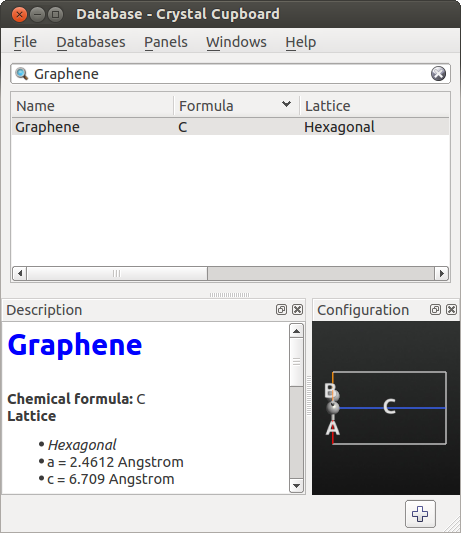The Script Generator should now have the following settings:Now open theNew Calculator block and make the following changes:

• Select the ATK-SE: Slater-Koster calculator.
• Change the k-point sampling to (5, 5, 1).
• Go to the Slater-Koster basis set, and check that the installed basis sets are in the basis set list. Select the “DFTB [mio]” basis set.

Note

If you do not see the “DFTB [mio]” basis set in the list, something went wrong in the installation process. Check that the file atkpython/share/tightbinding/dftb/mio/C-C.skf exists in your ATK installation directory.

• Uncheck the No SCF iteration box, in order to invoke a self-consistent DFTB calculation.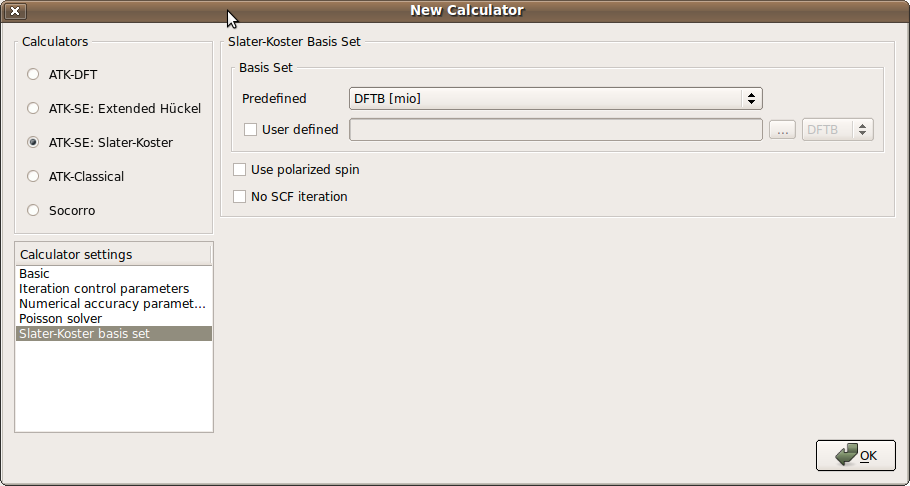Attention

The default assumption is that a tight-binding model is non-self-consistent. It is the responsibility of the user to uncheck the No SCF iteration box for models which are to be self-consistent. Most DFTB models are self-consistent.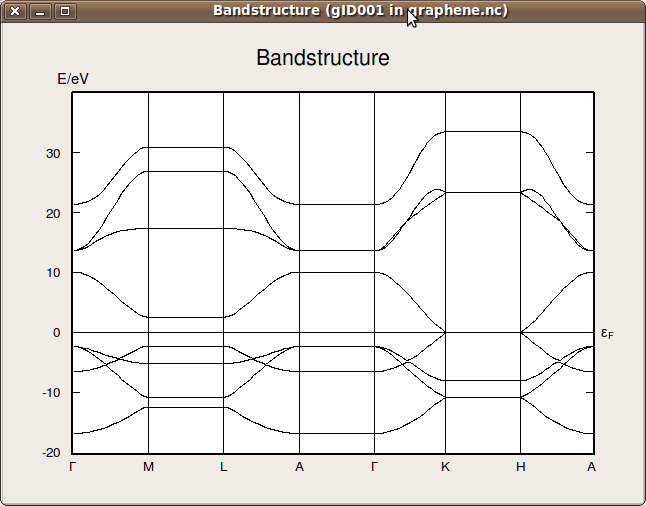Note

If the test went well, the DFTB parameters are properly installed. You are then able to use DFTB parameters instead of DFT for some of the other ATK tutorials available on the QuantumWise website (including quantum transport calculations), in order to save computational time.

Obviously, this only works if the elements used are covered by the DFTB basis sets.

## Spin polarized calculations with DFTB¶

Calculating the spin polarization of a graphene ribbon

In the following you will set up a graphene nanoribbon, and perform a spin-polarized calculation using the DFTB [mio] model.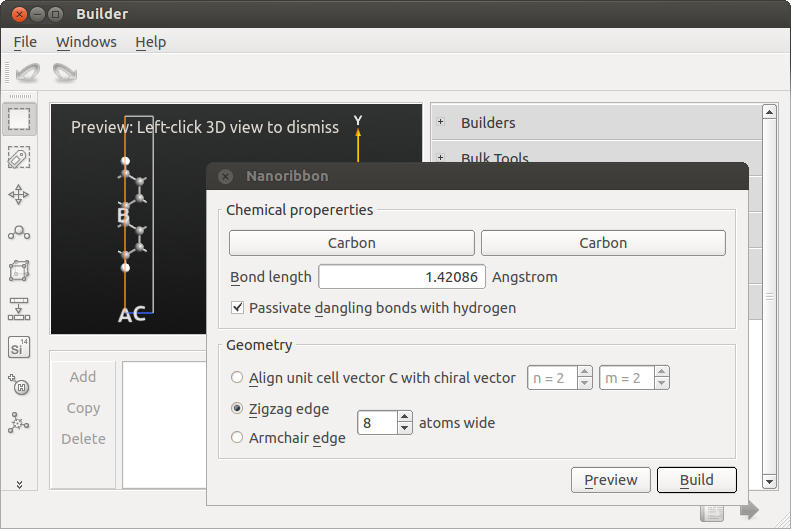To set up a calculation of the band structure, send the structure to the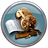Script Generator using the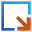button in the lower right-hand corner of the builder window.

In the Script Generator, add the following blocks:

and change the output filename to ribbon.nc.

The Script Generator should now have the following settings: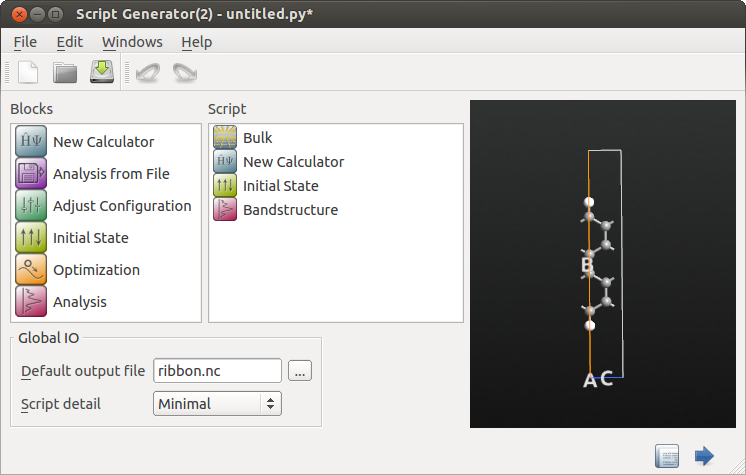Open theNew Calculator block.

• Select the ATK-SE: Slater-Koster calculator.
• Change the k-point sampling to (1,1,11).
• Go to the Slater-Koster basis set and select the “DFTB [mio]” basis set.
• Check the Use polarized spin box, in order to perform a spin-polarized DFTB calculation.

Note

The DFTB parameter set does not include spin polarization parameters. Instead, spin polarization parameters are obtained from the ATK_W database.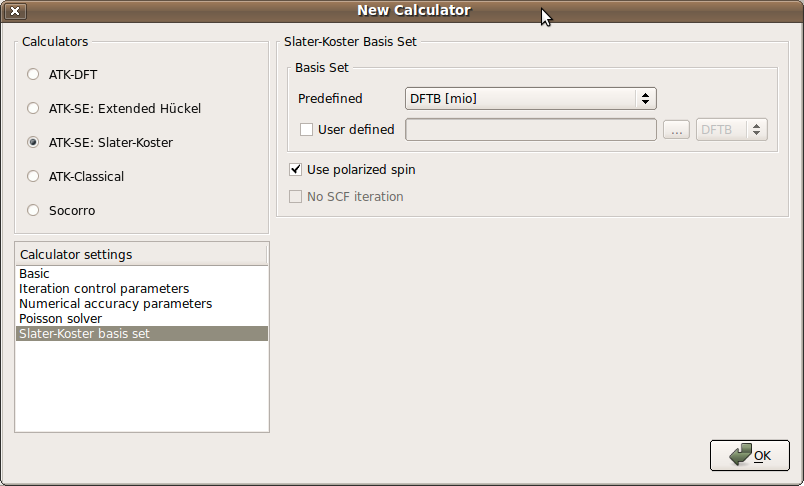Finish by clicking OK.

Next, set up the initial spin state of the system:

• Open the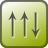Initial State block by double-clicking it.

• Set the Initial state type to User spin.

• Under Spin, set the default spin of both Carbon and Hydrogen to 0.

• In the 3D view:
• Click the upper carbon atom (index 0 in the list of atoms) and set its initial relative spin to 1.0.
• Repeat this procedure for the lower carbon atom (number 1 in the list), setting its initial relative spins to -1.0. (Don’t be confused by the fact that the carbon atoms are not ordered by Y coordinate).
• Leave the hydrogen atoms and the other carbon atoms with initial relative spin values of 0.0.

The dialogue should now look as shown below.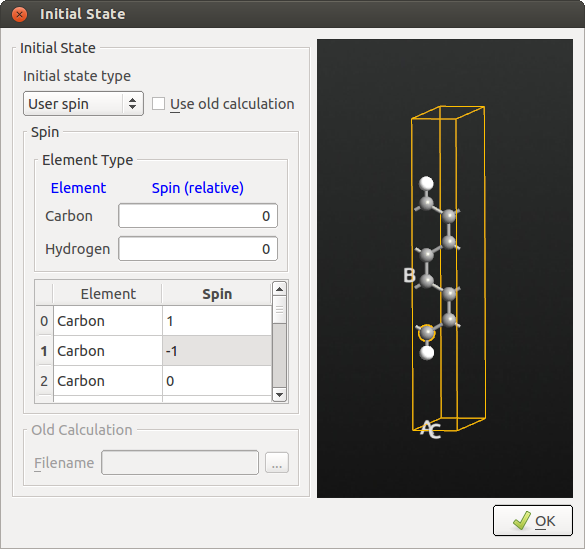Finally, open theBandstructure block:

• Change Points pr. segment to 200. This implies that each band will be calculated with a resolution of 200 points.
• Also notice that the default suggested route in the Brillouin zone (from the $$\Gamma$$-point G=(0,0,0) to Z=(0,0,1/2) in units of the reciprocal lattice vectors) is the appropriate one.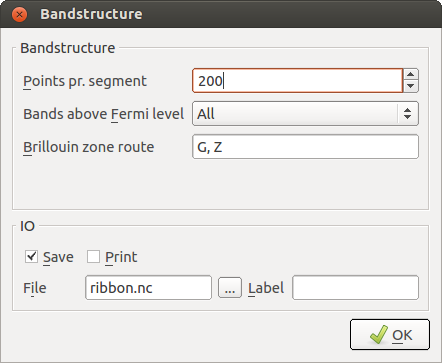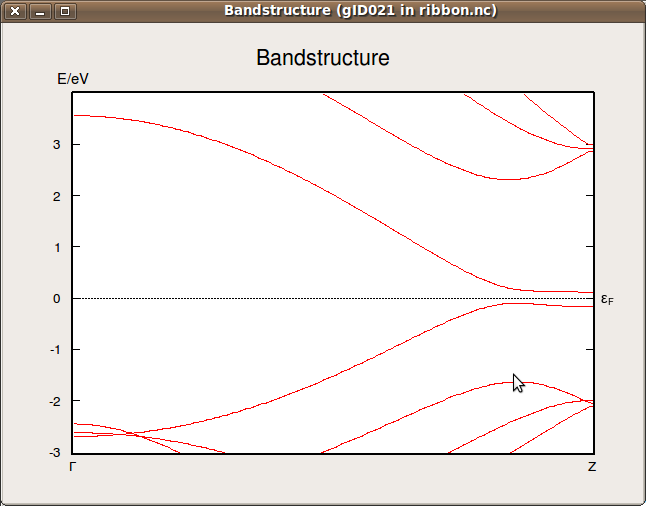Calculate the properties of a series of configurations using scripting

If you want to calculate the bandstructure of a number of nanoribbons, it is very convenient to use Python scripting. The script below performs a calculation of the bandstructure of nanoribbons with chiral index (n,n), where n runs from 1 to 10.

Attention

The chiral index notation for nanoribbons in ATK is based on the linear combination of the planar graphene unit vectors that are used to form the unit cell. Thus, (n,n) have zigzag edges, and actually correspond to an unrolled armchair nanotube with the same chiral indices.

For armchair edge nanoribbons, the chiral indices are (n,0).

# Setup the DFTB Calculator
basis_set = DFTBDirectory("dftb/mio/")
pair_potentials = DFTBDirectory("dftb/mio/")

numerical_accuracy_parameters = NumericalAccuracyParameters(
k_point_sampling=(1, 1, 51),
)

iteration_control_parameters = IterationControlParameters()

calculator = SlaterKosterCalculator(
basis_set=basis_set,
pair_potentials=pair_potentials,
numerical_accuracy_parameters=numerical_accuracy_parameters,
iteration_control_parameters=iteration_control_parameters,
spin_polarization=True,
)

#loop over different NanoRibbons
for n in range(1,10):
#generate the nanoribbon
bulk_configuration = NanoRibbon(n,n)

#Determine the initial spin of the configuration
elements = bulk_configuration.elements()
coords = bulk_configuration.fractionalCoordinates()
scaled_spins = numpy.zeros(len(elements))

#find the index of the two edge Carbon atoms,
ymin = 0.5
ymax = 0.5
imin=0
imax=0
for i in range(len(elements)):
if coords[i] < ymin and elements[i] == Carbon:
ymin = coords[i]
imin = i
if coords[i] > ymax and elements[i] == Carbon:
ymax = coords[i]
imax = i

# set opposite spins on the edge Carbon atoms
scaled_spins[imin] = 1
scaled_spins[imax] = -1

#attach calculator, and set the initial spin
bulk_configuration.setCalculator(
calculator(),
initial_spin=InitialSpin(scaled_spins=scaled_spins),
)
bulk_configuration.update()

# Calculate Bandstructure
bandstructure = Bandstructure(
configuration=bulk_configuration,
route=['G', 'Z'],
points_per_segment=200,
bands_above_fermi_level=All
)
nlsave('bandgap.nc',bandstructure)


w_list = []
gap0_list = []
gap1_list = []
# read a list with the bandstructure data
bandstructure = nlread('bandgap.hdf5', Bandstructure)

for i in range(len(bandstructure)):
# get all the bandlines
energies = bandstructure[i].evaluate().inUnitsOf(eV)
#calculate the number of occupied bands
occupied_bands = numpy.sum(numpy.array(energies) < 0)
#calculate the minimum direct band gap
gap0 = numpy.min(energies[:,occupied_bands]- energies[:,occupied_bands-1])
#calculate the band gap at the zone boundary
gap1 = energies[-1,occupied_bands]- energies[-1,occupied_bands-1]
#determine the coordinates in the y direction
y_values = NanoRibbon(i+1,i+1).cartesianCoordinates().inUnitsOf(Ang)[:,1]
# calculate the width, as distance between furthest carbon atoms
# i.e. calculate the  H-H distance and subtracting the C-H distance
w = numpy.max(y_values)-numpy.min(y_values)-2.2
#append calculated values to the list
w_list.append(w)
gap0_list.append(gap0)
gap1_list.append(gap1)

#print the data
import pylab
pylab.figure()
pylab.plot(w_list,gap0_list, 'k-o')
pylab.plot(w_list,gap1_list, 'k-o', markerfacecolor='white')
pylab.xlabel(r"$w_z$ ($\AA$)", size=16)
pylab.ylabel("$\Delta_z$ (eV)", size=16)
pylab.show()Fig. 25 Direct band gap (solid circles) and the band gap at the zone boundary as function of the width of the nanoribbon.

Attention

Except for the smallest ribbon, the plot shows the same qualitative behavior as Fig. 4c in Ref. . The discrepancy in the results are related to the accuracy of the DFTB method compared with a DFT-LDA calculation. To test this, you can modify the script and change the definition of the calculator:

calculator = LCAOCalculator(
numerical_accuracy_parameters=numerical_accuracy_parameters,
exchange_correlation = LSDA.PZ
)


Remember to also modify the name of the output file in order to separate the new data from the old data. Then execute the script.

References

  Elstner, D. Porezag, G. Jungnickel, J. Elsner, M. Haug, T. Frauenheim, S. Suhai, and G. Seifert, Phys. Rev. B 58, 07260 (1998)
  Kohler, T. Frauenheim, B. Hourahine, G. Seifert, and M. Sternberg, J. Phys. Chem. A 111, 5622 (2007)
  Y.W. Son, M.L. Cohen, and S.G. Louie, Phys. Rev. Lett. 97, 216803 (2006)